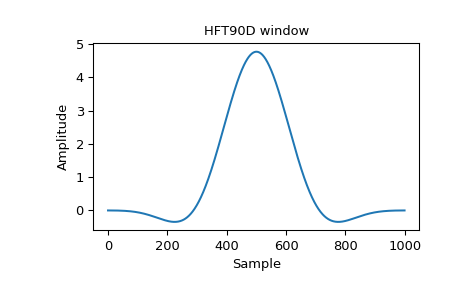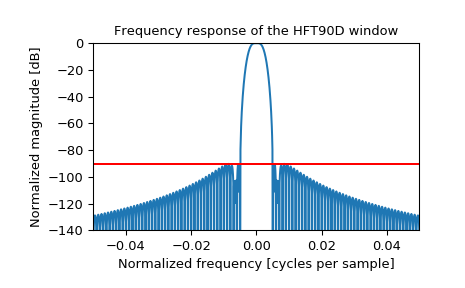# scipy.signal.windows.general_cosine¶

scipy.signal.windows.general_cosine(M, a, sym=True)[source]

Generic weighted sum of cosine terms window

Parameters
Mint

Number of points in the output window

aarray_like

Sequence of weighting coefficients. This uses the convention of being centered on the origin, so these will typically all be positive numbers, not alternating sign.

symbool, optional

When True (default), generates a symmetric window, for use in filter design. When False, generates a periodic window, for use in spectral analysis.

References

1

A. Nuttall, “Some windows with very good sidelobe behavior,” IEEE Transactions on Acoustics, Speech, and Signal Processing, vol. 29, no. 1, pp. 84-91, Feb 1981. DOI:10.1109/TASSP.1981.1163506.

2(1,2)

Heinzel G. et al., “Spectrum and spectral density estimation by the Discrete Fourier transform (DFT), including a comprehensive list of window functions and some new flat-top windows”, February 15, 2002 https://holometer.fnal.gov/GH_FFT.pdf

Examples

Heinzel describes a flat-top window named “HFT90D” with formula: 

$w_j = 1 - 1.942604 \cos(z) + 1.340318 \cos(2z) - 0.440811 \cos(3z) + 0.043097 \cos(4z)$

where

$z = \frac{2 \pi j}{N}, j = 0...N - 1$

Since this uses the convention of starting at the origin, to reproduce the window, we need to convert every other coefficient to a positive number:

>>> HFT90D = [1, 1.942604, 1.340318, 0.440811, 0.043097]


The paper states that the highest sidelobe is at -90.2 dB. Reproduce Figure 42 by plotting the window and its frequency response, and confirm the sidelobe level in red:

>>> from scipy.signal.windows import general_cosine
>>> from scipy.fftpack import fft, fftshift
>>> import matplotlib.pyplot as plt

>>> window = general_cosine(1000, HFT90D, sym=False)
>>> plt.plot(window)
>>> plt.title("HFT90D window")
>>> plt.ylabel("Amplitude")
>>> plt.xlabel("Sample")

>>> plt.figure()
>>> A = fft(window, 10000) / (len(window)/2.0)
>>> freq = np.linspace(-0.5, 0.5, len(A))
>>> response = np.abs(fftshift(A / abs(A).max()))
>>> response = 20 * np.log10(np.maximum(response, 1e-10))
>>> plt.plot(freq, response)
>>> plt.axis([-50/1000, 50/1000, -140, 0])
>>> plt.title("Frequency response of the HFT90D window")
>>> plt.ylabel("Normalized magnitude [dB]")
>>> plt.xlabel("Normalized frequency [cycles per sample]")
>>> plt.axhline(-90.2, color='red')
>>> plt.show()